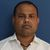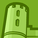# QlikView Documents

QlikView documentation and resources.

Announcements
Document boards are being consolidated, this board no longer allows NEW documents READ MORE

# Date Level Analysis - WTD, MTD, QTD & YTD (Current Year & Previous Year)

cancel
Showing results for
Did you mean:Specialist III

## Date Level Analysis - WTD, MTD, QTD & YTD (Current Year & Previous Year)

Hello Friends,

WTD (Week To Date), MTD(Month To Date), QTD (Quarter To Date) & YTD(Year To Date) are commonly used for any kind of analysis. Below are some expression which you can use while doing the same:

Very important to have the DateKey field in number format by using Floor(Date) AS DateKey. This will gives you numeric equivalent of the respective date. Post that you can derive the required expressions. In this case I have created expressions for WTD, MTD, QTD & YTD for current year as well as previous year. Also included Rolling MTD for 12 months.

WTD

//Current Year

Sum({<Year=, Quarter=, Month=, Week=, [Order Date]=, DateKey={">=\$(=Num(WeekStart(Max(DateKey)))) <=\$(=Max(DateKey))"}>}Sales)

//Previous Year

Sum({<Year=, Quarter=, Month=, Week=, [Order Date]=, DateKey={">=\$(=Num(WeekStart(Max(DateKey),-52))) <=\$(=AddYears(Max(DateKey),-1))"}>}Sales)

MTD

//Current Year

Sum({<Year=, Quarter=, Month=, Week=, [Order Date]=, DateKey={">=\$(=Num(MonthStart(Max(DateKey)))) <=\$(=Num(Max(DateKey)))"}>}Sales)

//Previous Year

Rolling MTD

//Current Month

Sum({<Year=, Quarter=, Month=, Week=, [Order Date]=, DateKey={">=\$(=Num(MonthStart(Max(DateKey)))) <=\$(=Num(Max(DateKey)))"}>}Sales)

//Current Month - 1

//Current Month - 2

.

.

.

//Current Month - 11

QTD

//Current Year

Sum({<Year=, Quarter=, Month=, Week=, [Order Date]=, DateKey={">=\$(=Num(QuarterStart(Max(DateKey)))) <=\$(=Max(DateKey))"}>}Sales)

//Previous Year

YTD

//Current Year

Sum({<Year=, Quarter=, Month=, Week=, [Order Date]=, DateKey={">=\$(=Num(YearStart(Max(DateKey)))) <=\$(=Max(DateKey))"}>}Sales)

//Previous Year

References:

https://community.qlik.com/docs/DOC-6163

I learned all these by referring documents & discussions generated by all Qlik Community members. Thank so much all of you!

Hope this will be of help.

Regards!

Rahul

Tags (1)
Labels (5)

• ### QlikView ApplicationsPartner

Good information. Thank you.Not applicable

Hi,

Can someone please explain the DateKey and Floor aspect of this to me?

My Date field in my data model is called DATE but Floor(DATE) gives me and error

Also what would I replace [Order Date] with?Specialist III

Hello Nicholas,

Trust that you are doing well.

I have used the DateKey field in number format by using Floor([Order Date]). This returned me numeric equivalent of the respective date. I used this to apply date range validation with the help of equivalent number.

//By Replacing [Order Date]

Sum({<Year=, Quarter=, Month=, Week=, [Order Date]={">=\$(=YearStart(Max([Order Date]))) <=\$(=Max([Order Date]))"}>}Sales)

If your expression is still throwing an error then please share the application with sample data. This will help me to provide you desired response.

Regards!

RahulPartner

Hi Nicholas,

Don't panic, set expressions seems difficult at first sight, but then you'll love working with them.

Let's suppose you have a date field (you don't have to create variables); only a date field will do the job.

And let's suppose you've created a calendar in your script so you have Year,Month, Quarter and Week fields

YTD: if we select 12/04/2016: YTD will return our measure from 01/01/2016 to 12/04/2016

How we do that?

Suppose our measure is : sum(Sales)

1)First changes: sum({<date=,Year=,Month=,Quarter=>}Sales)

We add these to force Qlik to not take into consideration our selection of date for example.

Let me explain in better words, if you don't write the "date=" and select the date 12/04/2016; Qlik will filter all the data to that selected point and then return the sum(Sales) for the day 12/04/2016.

To prohibit this, we must write the date=.

2) Second change: sum({<date=,Year=,Month=,Quarter=, date={">=\$(=YearStart(Max(date)))<=\$(=Max(date))"}>}Sales)

Let explain this : date={">=\$(=YearStart(Max(date)))<=\$(=Max(date))"}

We want to work from date=01/01/2016 to the selected date=12/04/2016 right?

So we're working with the field :

a) date={    }

b) Now we wanna this date to be <=selected date which is max(date) ;

max(date) is a function so it needs an "=" sign:

=max(date)

when we have a '=' we add the \$ (before each calculation) : \$(=max(date) ) => this is 12/04/2016

Now we add the <= so we'll have :  <=\$(=max(date) )

for the second part, we want our date to be >=01/01/2016 which is the start of the year:

a) same approach, we use the YearStart function that returns the start of the selected year: >=\$(=YearStart(Max(date)))

Now our expression is : from : date={    }

to : date={>=\$(=YearStart(Max(date)))<=\$(=Max(date))}

Now, in a set expression, if we wanna work with the year 2016 for example which is numeric: we call it without quotes:

Year={2016}

If we wanna focus on a country, Tunisia for example which is a string: we call it with quotes

Country={'Tunisia'}

In our case, we focusing on a range of dates created by an expression, so we surround it by double quotes:

date={">=\$(=YearStart(Max(date)))<=\$(=Max(date))"}

Final expression for YTD:

sum({<date=,Year=,Month=,Quarter=, date={">=\$(=YearStart(Max(date)))<=\$(=Max(date))"}>}Sales)

Same approach for MTD:

sum({<date=,Year=,Month=,Quarter=, date={">=\$(=MonthStart(Max(date)))<=\$(=Max(date))"}>}Sales)

Hope this helps,

Omar,Creator III

Hi,

If I use date, Year, Month, Quarter columns as dimension, then my MTD, YTD or WTD values will be wrong? Or should I consider those columns as dimension?

Thanks,

SandipContributor III

Hello,

This is very helpful, I just have follow-up question. How will we implement this in a selection box for example wherein the options will be MTD, QTD, YTD and whichever is selected will affect the calculations on the charts?

Thanks,

RoebrichSpecialist III

Hello Roebrich,

Apologies for the delay in response.

Considering the previously attached solution as base for your requirement, you can follow below steps:

1. Create an Island Table

AGGTYPE:

Type

WTD

MTD

QTD

YTD

];

2. Add List box to show Type. Select anyone of the value and set the Always One Selected Value option present on General tab.

3. Add Conditional Expression for Every expression

Week Expressions: =If(GetFieldSelections([Type])='WTD',1,0)

Month Expressions: =If(GetFieldSelections([Type])='MTD',1,0)

Quarter Expressions: =If(GetFieldSelections([Type])='QTD',1,0)

Year Expressions: =If(GetFieldSelections([Type])='YTD',1,0)

Hope this will help.

Regards!

Rahul PawarCreator

Thank you.Contributor III

One question though.

I tried this with a date filter but it give a value = 0.

For example if I select a date = January 2020 in the filter, it shows the current year with right values but shows 0 for the previous year.

Is it possible to make it dynamic(change depending on the date filter)?

Version history
Last update:
‎2017-01-24 07:32 AM
Updated by: